# Advanced Tutorial Lesson 3: Investigating Audio Power Amplifiers

 Tutorial Project: Investigating Audio Power Amplifiers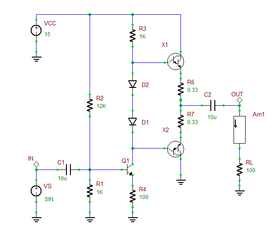Objective: In this project, you will analyze several BJT amplifiers and examine their power efficiency and distortion characteristics. Concepts/Features: Bipolar Junction Transistor Common Emitter Amplifier Class AB Amplifier Voltage Gain Load Current Power Efficiency Transient Test Darlington Pair Distortion Meter Total Harmonic Distortion Minimum Version Required: All versions

## What You Will Learn

In this tutorial you will build and test common emitter and class AB amplifiers and compare their power efficiencies. Since small-signal approximation does not hold well for power amplifiers, you will use RF.Spice's Transient Test to analyze these nonlinear circuits.

## Exploring a Basic Common-Emitter Amplifier

The following is a list of parts needed for this part of the tutorial lesson:

Part Name Part Type Part Value
VCC Voltage Source DC, 15
VS Voltage Source Waveform TBD
R1 Resistor 66k
R2 Resistor 6k
R3 Resistor 10k
R4 Resistor 900
RL Resistor 10k
C1 - C2 Capacitor 1u
C3 Capacitor 220n
Q1 Q2N3904 NPN BJT Defaults
Am1 Ammeter N/A
IN, OUT Voltage Probe Marker N/A

Place and connect the parts of the basic common emitter amplifier as shown in the figure below:

Set the waveform of the voltage source VS according to the following table:

 Offset Voltage 0 100mV 1kHz 0 0

First, run a DC Bias Test of your amplifier to find the operating point parameters of Q1. You will find ICQ = 755μA. Therefore, the small-signal transconductance of the transistor is gm = ICQ / VT = 0.029S. Run a Transient Test of this circuit with the parameters specified below:

 Start Time 5m 10m 10u 1u v(in), v(out), i(rl), p(vcc), p(rl)

Note that the start time of the transient test in this tutorial is t = 5ms. Before you start to sample the waveforms, you need to let the transients settle down. The simulation results are shown in the figure below. Note that the five plots involve voltages, currents and powers with entirely different ranges and scales. Therefore, you need to use the "Zoom to Selected Plot" feature of the graph window to better view the details of each plot.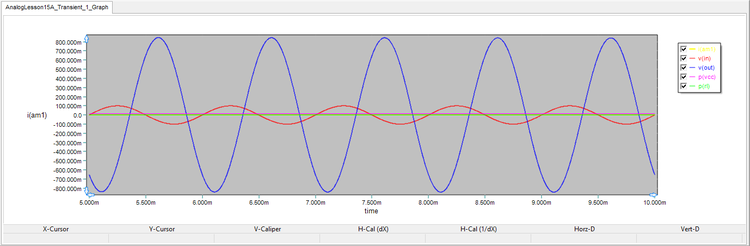The joint graph of v(in), v(out), i(rl), p(vcc) and p(rl) in the common emitter amplifier circuit zoomed to v(out).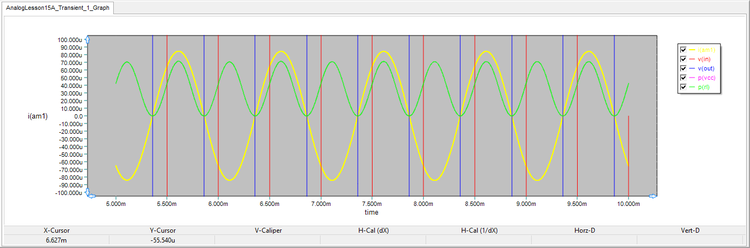The joint graph of v(in), v(out), i(rl), p(vcc) and p(rl) in the common emitter amplifier circuit zoomed to i(rl) and p(rl).

In the above figure, p(vcc) is the DC power of the DC power supply VCC, while p(rl) is the power delivered to the load. The ratio p(rl)/p(vcc) defines the power efficiency of the amplifier. From the above plots, you can read the peak-to-peak input and output voltages, voltage gain and power efficiency:

RL Vin(p-p) Vout(p-p) Voltage Gain IRL(p-p) PVCC PRL Power Efficiency
10kΩ 1686mV 200mV 8.43 168.5μA 15.68mW 71.28μW 0.46%

## Observing the Effect of Low Load Resistance

Audio power amplifiers are usually used to drive very small loads. Change the value of RL to 100Ω, and run the transient test once again. The results for a 100Ω load are shown ion the figure and summarized below.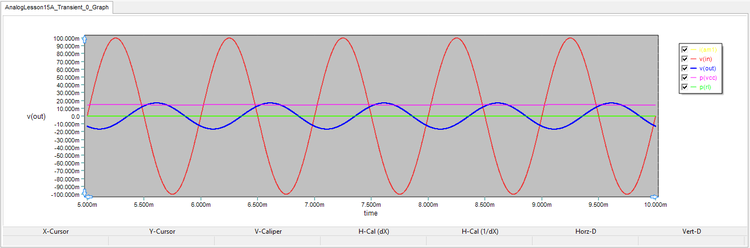The joint graph of v(in) and v(out) in the common emitter amplifier circuit with RL = 100Ω.
RL Vin(p-p) Vout(p-p) Voltage Gain IRL(p-p) PVCC PRL Power Efficiency
100Ω 34mV 200mV 0.17 167.4μA 14.46mW 2.8μW 0.02%

You can see that the peak-to-peak output voltage has dropped drastically to 34mV. This is obviously expected since the 100Ω load shunts the collector resistor R3 and reduces the voltage gain: GV = -RC,tot / RE,tot.

Finally, let's increase the input signal level from 100mV to 1V (2Vp-p) to see how the output is affected. The figure below shows the results: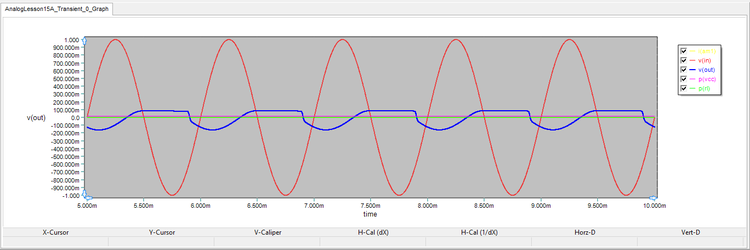Distorted output voltage due to large input voltage in the common emitter amplifier circuit.

You can see that the load current has increased significantly to more than 1mA but at the expense of output voltage distortion. You may conclude that the common emitter amplifier is not an effective solution when the load is small.

## Building and Testing a Class AB BJT Amplifier

The following is a list of parts needed for this part of the tutorial lesson:

Part Name Part Type Part Value
VCC Voltage Source DC, 15
VS Voltage Source Waveform TBD
R1 Resistor 1k
R2 Resistor 12k
R3 Resistor 1k
R4 Resistor 100
R5 - R6 Resistor 0.33
RL Resistor 100
C1 - C2 Capacitor 10u
D1 - D2 1N4148 Diode Defaults
Q1 - Q2 Q2N3904 NPN BJT Defaults
Q3 Q2N3906 PNP BJT Defaults
Am1 Ammeter N/A
IN, OUT Voltage Probe Marker N/A

Place and connect the part of the class AB BJT amplifier as shown figure below. The transistor Q1 acts as the driver amplifier. The complementary NPN-PNP pair Q2 and Q3 form the class AB amplifier, and the diodes D1 and D2 are used to bias them.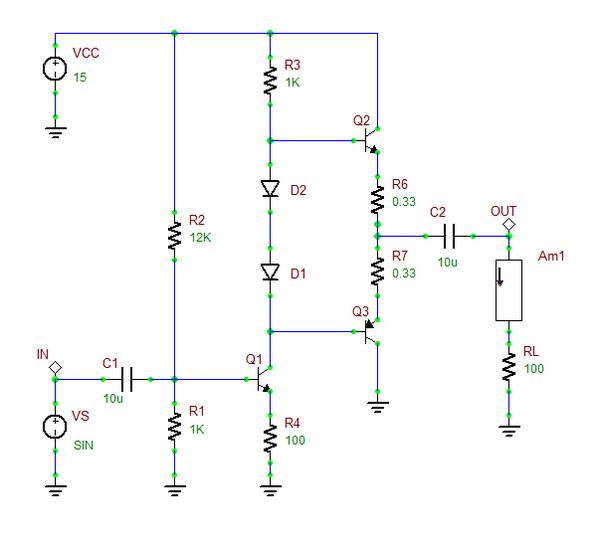The class AB BJT amplifier with a driver stage.

Set the waveform of the voltage source VS according to the following table:

 Offset Voltage 0 500mV 1kHz 0 0

First, run a DC Bias Test of your amplifier to find the operating point parameters of the three transistors. The results are given below. As you would expect, the collector currents of Q2 and Q3 are almost equal in magnitude and have opposite signs.

ICQ1 ICQ2 ICQ3
4.371mA 9.995mA -9.983mA

Run a Transient Test of this circuit with the parameters specified below:

 Start Time 5m 10m 10u 1u v(in), v(out), i(rl), p(vcc), p(rl)

The results are shown in the figures below and summarized in the accompanying table. Note that the peak-to-peak output voltage is 7.36V without any serious distortion. The load peak-to-peak current is 73mA. In particular, the power efficiency is more than 23%, a significant improvement over the common emitter amplifier of the previous part.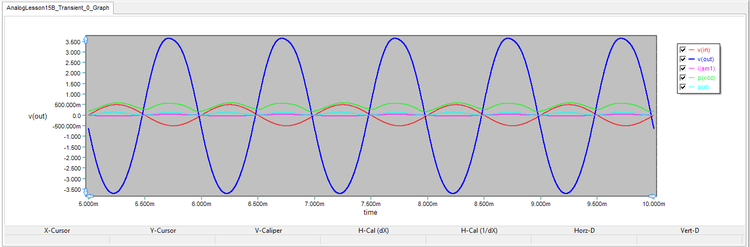The joint graph of v(in), v(out), i(rl), p(vcc) and p(rl) in the class AB amplifier circuit zoomed to v(out).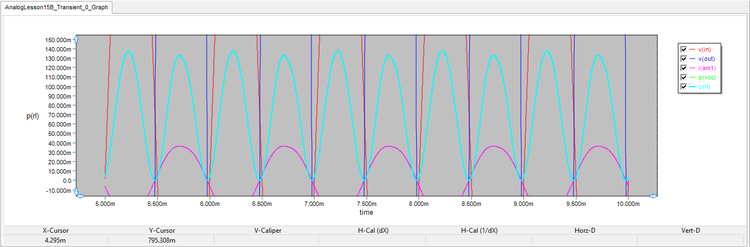The joint graph of v(in), v(out), i(rl), p(vcc) and p(rl) in the class AB amplifier circuit zoomed to i(rl) and p(rl).
RL Vin(p-p) Vout(p-p) Voltage Gain IRL(p-p) PVCC PRL Power Efficiency
100Ω 1000mV 7356mV 7.36 73mA 590mW 138mW 23.4%

Similar to the previous part of this lesson, next increase the input signal level to 1V (2Vp-p) and run a new transient test with the same parameters. This time, both the output voltage and current are highly distorted as shown in the figures below: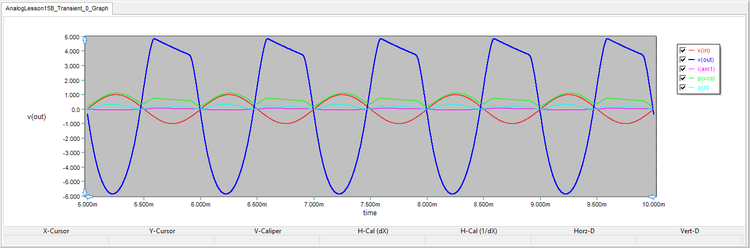Distorted output voltage due to large input voltage in the class AB amplifier circuit.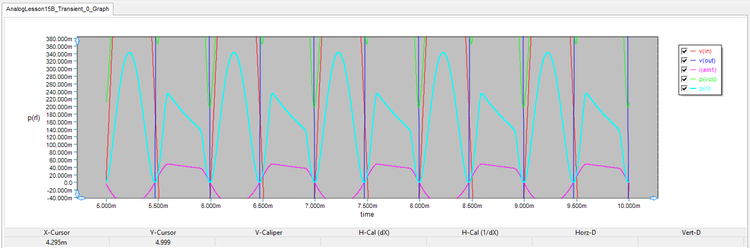Distorted load current and output power due to large input voltage in the class AB amplifier circuit.

## Distortion Analysis of the Class AB Amplifier

In Tutorial Lessons 13 and 14, you performer a "Fourier Analysis" of your circuit to characterize the spectral contents of signals. RF.Spice A/D also provides a convenient virtual instrument called Distortion Meter for this purpose. The distortion meter is particularly useful for the study of power amplifiers. To initiate a distortion meter, click the Add Meter button at the top of the "Virtual Instrument Panel" at the right side of the screen. From the drop-down menu, select Distortion Meter. Click the Setup button to open the property dialog of the instrument. It has three tabs. In the first tab labeled "Test Setup" set the circuits nodes 1 and 13 for input and output, respectively. Set the Test Frequency to 1kHz and Signal Amplitude to 0.5V, which is the peak amplitude of your source signal. Press the Go button. A harmonic analysis is performed, and the results are shown in the small black display of the instrument. A Total Harmonic Distortion (THD) of 3.22% is reported on the panel.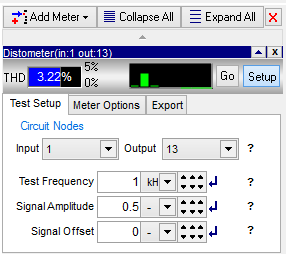The Test Setup tab of the Distortion Meter instrument panel.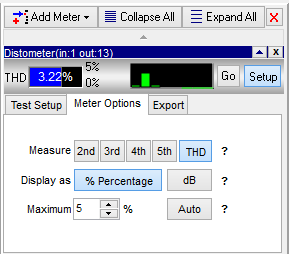The Meter Options tab of the Distortion Meter instrument panel.

You can see from the bar chart that your class AB BJT amplifier only has a third harmonic component at VS = 0.5V. In the second tab of the panel, you can select individual harmonics and measure their distortion as given in the table below:

2nd Harmonic Distortion 3rd Harmonic Distortion 4th Harmonic Distortion 5th Harmonic Distortion Total Harmonic Distortion (THD)
0.537% 3.11% 0.62% 0.128% 3.22%

## Exploring the Use of Darlington Pairs

The following is a list of the additional parts needed for this part of the tutorial lesson:

Part Name Part Type Part Value
X1 TIP141 NPN Darlington BJT Pair Defaults
X2 TIP147 PNP Darlington BJT Pair Defaults

In this part of the tutorial lesson, you will replace the complementary BJT pair Q2 and Q3 with complementary NPN and PNP Darlington pairs. The NPN X1 pair will replace Q2 and the PNP X2 pair will replace Q3 in the circuit of the previous part. Everything else will remain the same.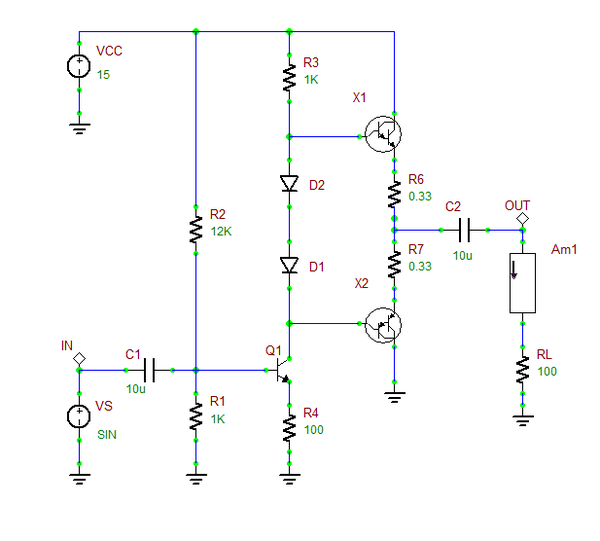The class AB BJT amplifier with complementary Darlington pairs and a driver stage.

Set the waveform of the voltage source VS according to the following table:

 Offset Voltage 0 500mV 1kHz 0 0

First, run a DC Bias Test of your amplifier to find the operating point parameters of all the transistors. The results are given below. Note that the DC currents passing through resistors R6 and R7 are the same as the emitter currents of the Darlington pairs.

ICQ1 IR6 IR7
4.366mA 31.756mA 31.756mA

Run a Transient Test of this circuit with the parameters specified below:

 Start Time 5m 10m 10u 1u v(in), v(out), i(rl), p(vcc), p(rl)

The results are shown in the figure and table below. You can see that both the load voltage and load current have increased in this case, which means a higher load power. In fact, the power delivered to the 100Ω load has increased by almost 50% over the previous case to more than 200mW. However, the power efficiency of the power amplifier has dropped to below 10%.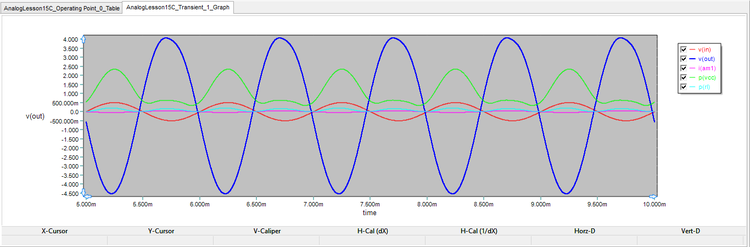The joint graph of v(in), v(out), i(rl), p(vcc) and p(rl) in the class AB amplifier circuit with complementary Darlington pairs.
RL Vin(p-p) Vout(p-p) Voltage Gain IRL(p-p) PVCC PRL Power Efficiency
100Ω 1000mV 8.6V 8.6 86mA 2.346W 205mW 8.7%

In the last part of this tutorial lesson, let's measure the distortion performance of your Darlington pair class AB amplifier. Follow the same procedure described above. The results are shown in the figures below: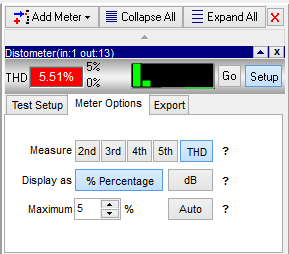The Test Setup tab of the Distortion Meter measuring a Darlington pair class AB amplifier.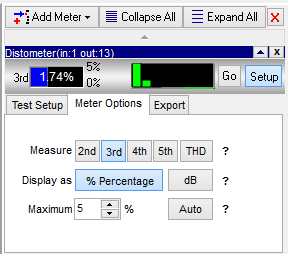The Meter Options tab of the Distortion Meter measuring a Darlington pair class AB amplifier.

Note that your Darlington pairs have introduced a significant second harmonic distortion, while the third harmonic distortion has decreased by half.

2nd Harmonic Distortion 3rd Harmonic Distortion 4th Harmonic Distortion 5th Harmonic Distortion Total Harmonic Distortion (THD)
5.2% 1.74% 0.454% 0.22% 5.51%